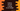# Python program to convert a string to an integer## How to convert a string to an integer in python :

In this tutorial, we will learn how to convert a string variable to an integer or a float in Python programming language. Python automatically assigns the data type according to the data stored in the variable. Sometimes we have to store a value as a string in a variable and then we need to calculate the sum of this variable with other numeric variables. In that case, we need to convert the string to an integer value (if we know that it will be integer always). For example, take a look at the below program : The first variable ‘firstnumber’_ is a string variable and the second variable ‘secondnumber’_ is an integer variable. The program is trying to add both of these variables.

But we can’t add a string variable with an integer. So, this program will throw one error like below : As you can see, the error is a ‘TypeError’ and the description of this error is that it can’t add one string object with an integer object. To fix this error, we will have to convert the string first_number to an integer variable.

This is the title of this tutorial and we will learn how to convert a number represented as a string to an integer. We will also learn what will happen if we can’t convert.

### Python program to convert a string to an integer :

The conversion is simpler than you think. Python has one built-in function called int() to make this conversion. Wrap the string value with int() and it will return the integer representation of the string.

The first variable is a string “1”. Using int() will convert it to an integer and we can add it to the other integer ‘2’. But, what will be the result if the string firstnumber_ holds a different value than a number like below : As you can see it throws one ‘ValueError’ with a message ‘invalid literal for int() with base ‘10’. ‘1@‘ is not a valid decimal number. Thus ‘int()’ method can’t convert it.

The best way to handle cases like this is to use one try-catch block like below : It will print the exception error.

Try-catch blocks are useful for handling code blocks that may throw an exception. It will first try to run the code defined inside the ‘try’ block. If an exception is raised, it will exit from the try block and run the code defined inside the catch block.

The catch block is normally used for printing out the error message to the developer. It makes debugging easy.

### Conclusion :

Converting a string to an integer is one of the most commonly faced problems in python development. For example, if your server is running on python and it is accepting integer values represented as String, we can use_ int()_ for the conversion.

But if it receives any other string values instead of an integer, the server will stop. As mentioned above, you can use try-catch and return one error message to the application in such scenarios.